## 6.3.7 HRLE

### 6.3.7.1 Outline of the node

This node estimates the stationary noise level using the Histogram-based Recursive Level Estimation (HRLE) method. HRLE calculates histograms (frequency distribution) of input spectra and estimates a noise level from the normalization accumulation frequency designated by the cumulative distribution and parameter $Lx$. A histogram is calculated with a previous input spectrum weighted with an exponent window, and the position of the exponent window is updated every frame.

### 6.3.7.2 Necessary files

No files are required.

### 6.3.7.3 Usage

When to use

This node is used when within to suppress noise using spectrum subtraction.

Typical connection

As shown in Figure 6.66, the input is connected after separation nodes such as GHDSS and the output is connected to the nodes that calculate an optimal gain such as CalcSpecSubGain . Figure 6.67 shows a connection example when EstimateLeak is used together.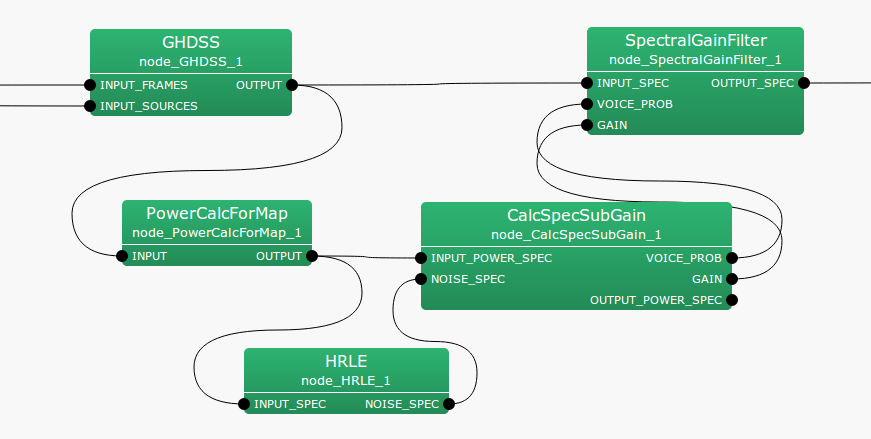Figure 6.66: Connection example of HRLE 1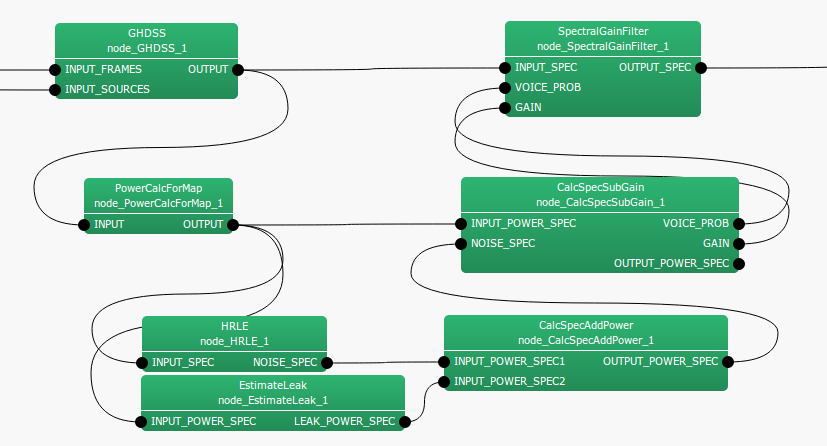Figure 6.67: Connection example of HRLE 2

### 6.3.7.4 Input-output and property of the node

Table 6.54: Parameter list of HRLE
 Parameter name Type Default value Unit Description LX 0.85 Normalization accumulation frequency ($Lx$ value). TIME_CONSTANT_METHOD LEGACY Time constant value definition. TIME_CONSTANT [pt] Time constant. DECAY_FACTOR [ms] Time constant. ADVANCE 160 [pt] Shift length of a frame. SAMPLING_RATE 16000 [Hz] Sampling frequency. NUM_BIN 1000 Number of bins of a histogram. MIN_LEVEL -100 [dB] The minimum level of a histogram. STEP_LEVEL 0.2 [dB] Width of a histogram bin. DEBUG false Debugging mode.

Input

INPUT_SPEC

: Map<int, ObjectRef>  type. Power spectrum of input signal.

Output

NOISE_SPEC

: Map<int, ObjectRef>  type. Power spectrum of estimated noise.

Parameters

LX

: float  type. Normalization accumulation frequency on an accumulation frequency distribution is designated in the range from 0 to 1. When designating 0, the minimum level is estimated. When designating 1, the maximum level is estimated. Median is estimated when 0.5 is designated. The default value is 0.85.

TIME_CONST_METHOD

: string  type. Time constant value definition. "LEGACY" uses time constant value in time sample unit,"MILLISECOND" uses time constant value in milliseconds. The default value is LEGACY.

TIME_CONSTANT

: float  type. A time constant (more than zero) is designated in time sample unit.

DECAY_FACTOR

: int  type. A time constant (more than zero) is designated in milliseconds.

: int  type. Shift length of a frame [samples], which must be equal to the values at a preceding node (e.g. AudioStreamFromMic or the MultiFFT ). The default value is 160.

SAMPLING_RATE

: int  type. Sampling frequency of the input waveform [Hz]. The default value is 16000.

NUM_BIN

: float  type. Designate the number of bins of a histogram. The default value is 1000.

MIN_LEVEL

: float  type. Designate the minimum level of a histogram in dB. The default value is -100.

STEP_LEVEL

: float  type. Designate a width of a bin of a histogram in dB. The default value is 0.2.

DEBUG

: bool  type. Designate the debugging mode. The default value is false. When it is set to true, the values of the cumulative histgram are output every 100 frames in the comma-separated text file format to the standard output. Output values are in the complex matrix value format with multiple rows and columns. The rows indicate positions of frequency bins and columns indicate positions of histograms. Each element indicates the complex values separated with parenthesis (right side is for real numbers and left side is for imaginaries). (Since the cumulative histogram is expressed with real numbers, and imaginary parts are usually 0. However, it does not necessarily mean that it will be 0 in future versions.) The additional value of a cumulative histogram for one sample is not 1 and they increase exponentially (for speedup). Therefore, note that cumulative histogram values do not indicate accumulation frequency itself. Most of the cumulative histogram values in each row are 0. When values are contained only in the positions that are close to the last column, the input values are great, exceeding the level range of the set histogram (overflow status). Therefore, part or all of NUM_BIN, MIN_LEVEL and STEP_LEVEL must be set to high values. On the other hand, when most of the cumulative histogram values of each row are constant values and different low values are contained only in the positions that are close to the first column, the input values are small below the level range of the set histogram (underflow status). Therefore, MIN_LEVEL must be set to low values.
Example of the output: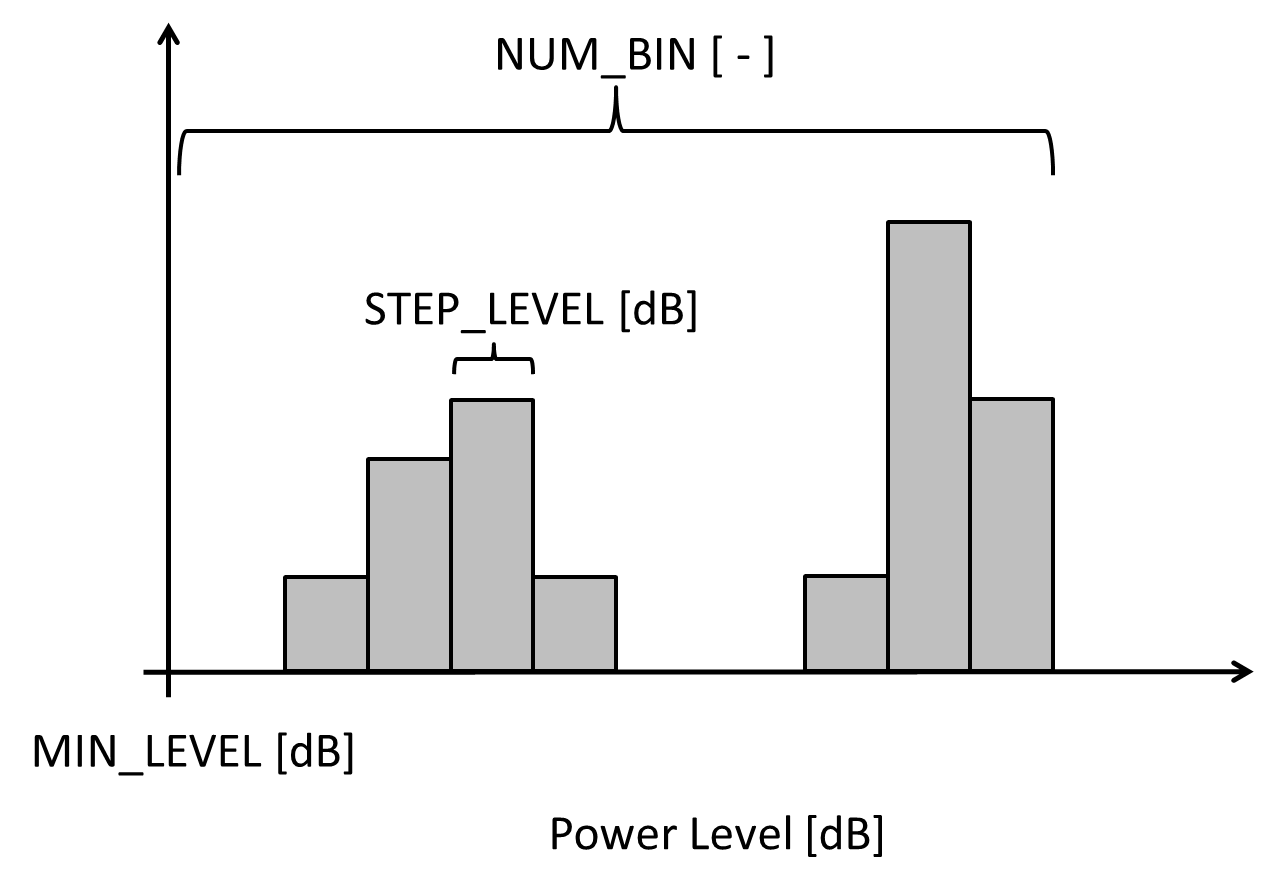Figure 6.68: Prameters (NUM_BIN, MIN_LEVEL, STEP_LEVEL)

---------- Compmat.disp()
----------
[(1.00005e-18,0), (1.00005e-18,0), (1.00005e-18,0), ...
, (1.00005e-18,0);
(0,0), (0,0), (0,0), ...
, (4.00084e-18,0);
...
(4.00084e-18,0), (4.00084e-18,0), (4.00084e-18,0), .., , (4.00084e-18,0)]
^T
Matrix size = 1000 x 257



### 6.3.7.5 Details of the node

Figure 6.69 shows a processing flow of HRLE. HRLE obtains a level histogram from the input power and estimates the $Lx$ level from the cumulative distribution. The $Lx$ level, as shown in Figure 6.70, is the level that normalization accumulation frequency in an accumulation frequency distribution becomes $x$. $x$ is a parameter. For example, when $x=0$, the minimum value is estimated, when $x=1$, maximum value is estimated and when $x=0.5$, a median is estimated in its processing.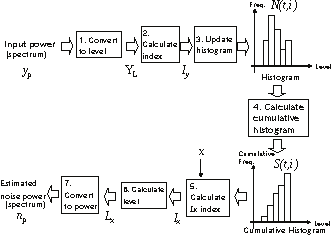Figure 6.69: Processing flow of HRLE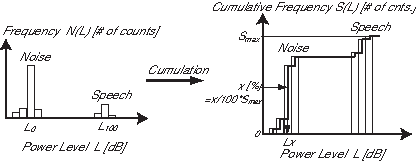Figure 6.70: Estimation of $Lx$ value

The details of the processing in HRLE are expressed by the following seven equations (corresponding to Figure 6.69). In the equations, $t$ indicates time (frame), $y_ p$ indicates input power (INPUT_SPEC) and $n_ p$ indicates estimated noise power (NOISE_SPEC]). $x$, $\alpha$, $L_{min}$ and $L_{step}$ are the parameters related to histograms and indicate normalization accumulation frequency (LX), time constant (TIME_CONSTANT), the minimum level (MIN_LEVEL) of a bin, and a level width (STEP_LEVEL) of a bin, respectively. $\lfloor a \rfloor$ indicates an integer most close to $a$ below $a$. Moreover, all variables except the parameters are functions of frequency and the same processing is performed independently for every frequency. In the equations, frequency is abbreviated for simplification.

 $\displaystyle Y_ L(t)$ $\displaystyle =$ $\displaystyle 10 \log _{10} y_ p(t), \label{eqn:revcon1}$ (71) $\displaystyle I_ y(t)$ $\displaystyle =$ $\displaystyle \lfloor (Y_ L(t)- L_{min})/ L_{step} \rfloor , \label{eqn:revcon2}$ (72) $\displaystyle N(t, l)$ $\displaystyle =$ $\displaystyle \alpha N(t-1, l)+ (1 - \alpha )\delta (l - I_ y(t)), \label{eqn:hitso}$ (73) $\displaystyle S(t, l)$ $\displaystyle =$ $\displaystyle \sum _{k=0}^ l N(t, k), \label{eqn:cumul}$ (74) $\displaystyle I_ x(t)$ $\displaystyle =$ $\displaystyle \mathop {\rm argmin}_ I \left[ S(t, I_{max}) \frac{x}{100} - S(t, I) \right], \label{eqn:search}$ (75) $\displaystyle L_ x(t)$ $\displaystyle =$ $\displaystyle L_{min} + L_{step} \cdot I_ x(t),$ (76) $\displaystyle n_ p(t)$ $\displaystyle =$ $\displaystyle 10^{L_ x(t)/10}$ (77)

### 6.3.7.6 References

(1) H.Nakajima, G. Ince, K. Nakadai and Y. Hasegawa: “An Easily-configurable Robot Audition System using Histogram-based Recursive Level Estimation”, Proc. of IEEE/RSJ Int. Conf. on Intelligent Robots and Systems (IROS), 2010 (to be appeared).